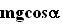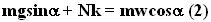## Tuesday, August 14, 2007

### Irodov Problem 1.80If there were no friction, as the system is accelerated towards the left, the mass on the inclined plane would climb up the inclined plane. However, the presence of friction binds them together and moves the mass towards the left along with the inclined plane. After a point, when the friction is not sufficient to hold the mass anymore, it will start to climb up the plane.
Let the mass be m and let the normal reaction offered from the inclined plane to the mass be N.

Forces on the mass m:
Let us resolve the forces acting on the mass in directions parallel and perpendicular to the surface of the inclined plane. Along the direction normal to the plane, there are two forces acting, i) the component of gravityand ii) the normal reaction from the surface N. Since the entire system accelerates towards the left with an acceleration w, the component of acceleration along the normal direction is given byas shown in the figure. Thus we have,In the direction parallel to the inclined plane there are two forces acting on the mass, i) the force of friction opposing the slipping of the mass upwards relative to the inclined plane Nk and ii) the component of gravitypulling it down the inclined plane. The component of acceleration of the mass, as shown in the figure is given by. Thus we have,Now we can solve for w from (1) and (2) to get,